# Multiple line graph with bar graph

I am using the grouped bar + line option to graph 3 different columns, currently the problem I am having is that I cannot move the bar and the line graph to the left axis and have the 2nd line graph stay on the right axis. I am trying to move the circled line to left axis.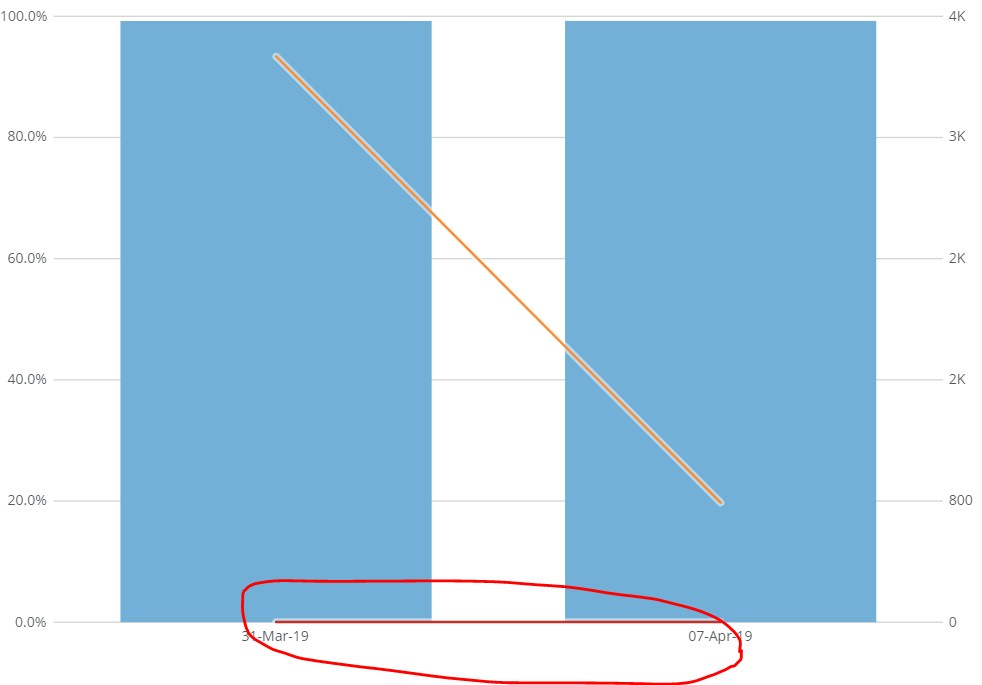Any insights?

Thanks

• Hi @hzeybek ,

So if I'm understanding your question correctly, it sounds like you have two columns that you want to be bars, and one column to be a line. I think you might want to use another visualization.

I have the Line + Grouped and Stacked Bar graph in my sample here. Whichever column you want to have as a line, put that in for the Y axis field. Then add your bar columns as series. Let me know if you had something else in mind!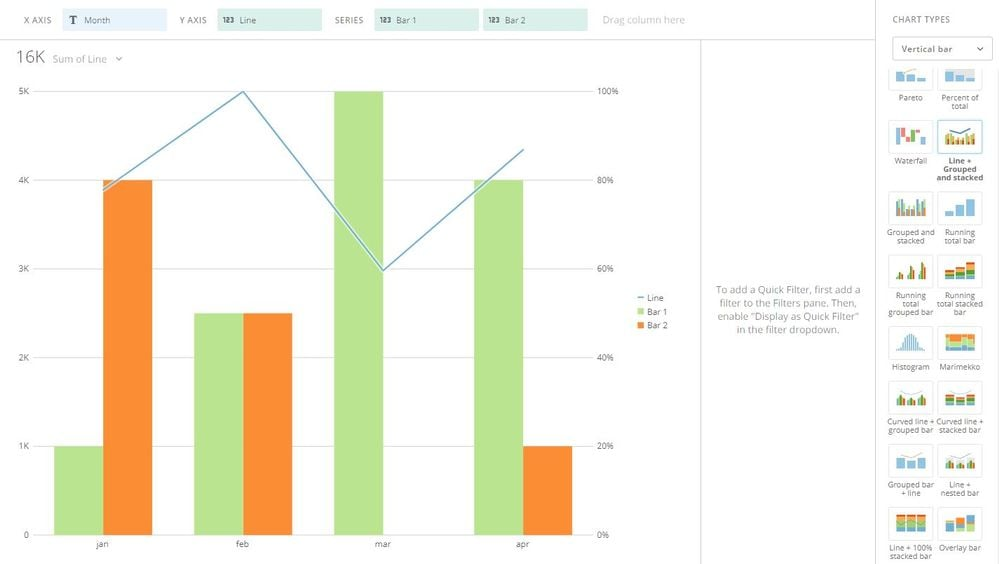EDIT: Sorry, just reread the subject line. Do you want the bar to remain a bar, and for the circled line to stay as a line? Both are showing percentages, correct?

•CoachIt's a setting under the "General" Chart properties.  You will need to have the two fields that you want to be lines added to your chart as the Y-axis and the first series.  Then the bar field (or fields) can be put as the second (or third, etc.) series.

You want to indicate that there should be 2 series on the left scale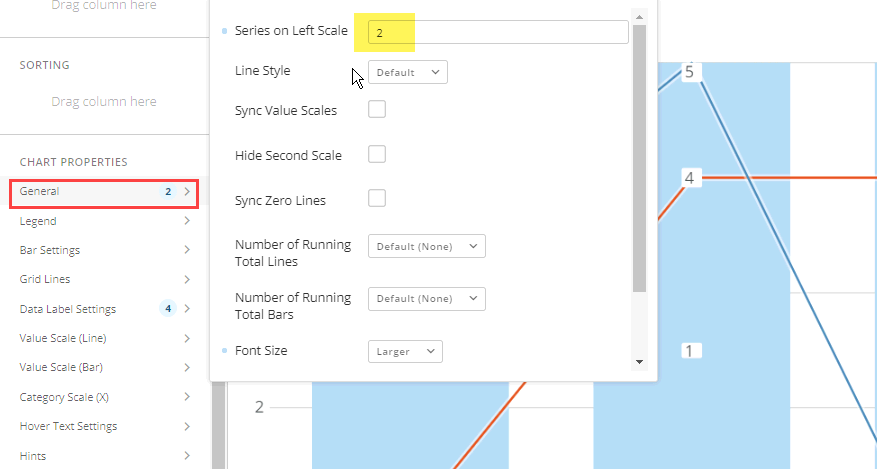“There is a superhero in all of us, we just need the courage to put on the cape.” -Superman
• Thanks for suggestions @Bolt , @ST_-Superman-_  but I am actually looking to have two line graphs overall but I need the one of the line graphs to be on the same axis as the bar graph, see below image from Excel.  The blue bar and the red line are both percentages which both of them are on the left axis, the orange is in units so it is on the right axis.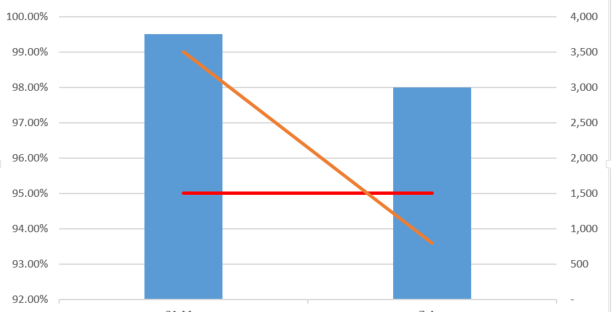Thanks

• @Bolt Just saw your edit, yes that is what I am looking for.  One caveat also is I need the percentages to be on the left axis.

Thanks

•CoachI would think that it is incredibly disorienting to see a graph with bars and multiple lines, all on different axis.

I think that best practice would be to at least keep all of the same element types on the same axis (lines on one axis, bars on the other). I would recommend evaluating the question you are trying to answer and what data elements are needed to get to the answer.  My assumption will be that we can find an easier way to lead the end user to the answer with a group of cards rather than trying to tie these elements together in a single view.

“There is a superhero in all of us, we just need the courage to put on the cape.” -Superman
• We are trying to show what the goal is in red line, the bars are how we performed and the other line is how many orders we have processed.  The idea is to see if we have a performance issue and is it related to the # of orders processed in one graph.

Thanks

•CoachAh. That makes more sense.  Have you tried using the "Scale Marker" chart property to display the goal?

“There is a superhero in all of us, we just need the courage to put on the cape.” -Superman
•Coach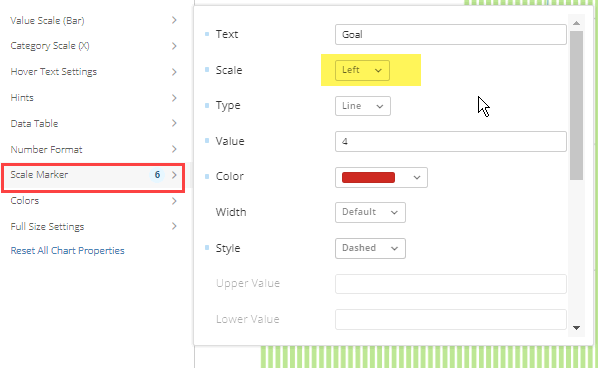“There is a superhero in all of us, we just need the courage to put on the cape.” -Superman
• Oh, didnt know about that option. Do you know if there is a way to set the value dynamicly, like to column name?

Thanks

•ContributorIf you can fix min and max of the two axis, you could multiply the target value by a factor to scale it so that it looks like it belongs to the left axis. If have done something similar in the past.

In a simple example, if your fixed axis are 0% to 100% and 0 to 4000. Multiplying a target of 80% by 4000 would give you a line that would be aligned with 80% on hte left axis

It would give out a bogus value if someonehovers hovers thier mouse over the line though.

This discussion has been closed.